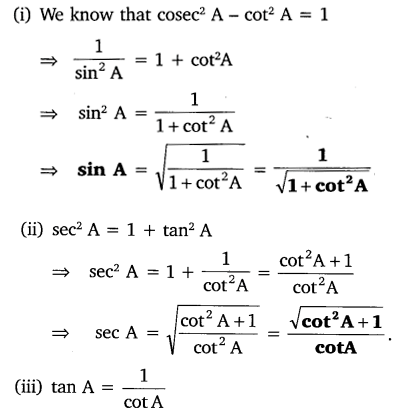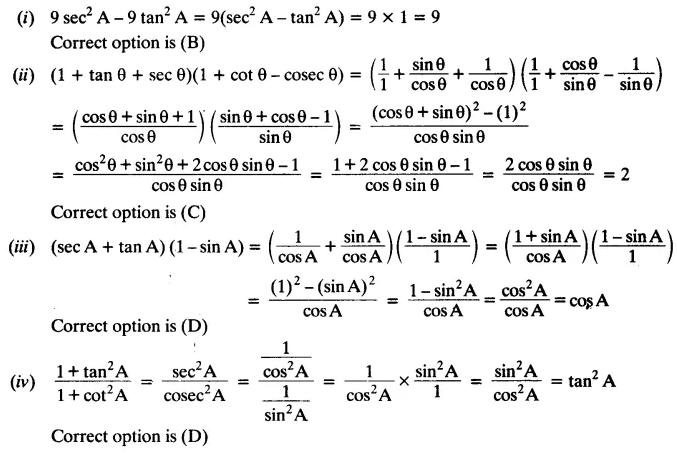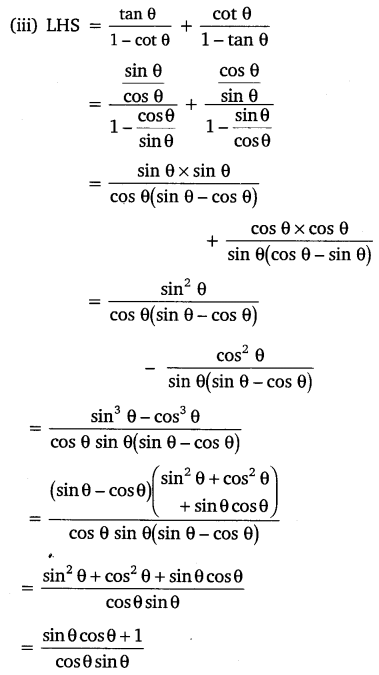# NCERT Solutions For Class 10 Maths Chapter 8 Introduction to Trigonometry Ex 8.4

Get Free NCERT Solutions for Class 10 Maths Chapter 8 Ex 8.4 Introduction to Trigonometry Class 10 Maths NCERT Solutions are extremely helpful while doing homework. Exercise 8.4 Class 10 Maths NCERT Solutions were prepared by Experienced ncert-books.in Teachers. Detailed answers of all the questions in Chapter 8 Maths Class 10 Coordinate Geometry Exercise 8.4 Provided in NCERT Textbook.NCERT Solutions For Class 10 Maths Chapter 8 Introduction to Trigonometry Ex 8.4

NCERT Solutions for Class 10 Maths Chapter 8 Introduction to Trigonometry Ex 8.4 are part of NCERT Solutions for Class 10 Maths. Here we have given NCERT Solutions for Class 10 Maths Chapter 8 Introduction to Trigonometry Ex 8.4.

Question 1.
Express the trigonometric ratios sin A, sec A and tan A in terms of cot A.
Solution:Question 2.
Write all the other trigonometric ratios of ∠A in terms of sec A.
Solution:Question 3.
Evaluate:Solution:Question 4.
Choose the correct option. Justify your choice.
(i) 9 sec² A – 9 tan² A = ……
(A) 1
(B) 9
(C) 8
(D) 0

(ii) (1 + tan θ + sec θ) (1 + cot θ – cosec θ) = ………..
(A) 0
(B) 1
(C) 2
(D) -1

(iii) (sec A + tan A) (1 – sin A) = ………….
(A) sec A
(B) sin A
(C) cosec A
(D) cos A

(iv)$\frac { 1+{ tan }^{ 2 }A }{ 1+cot^{ 2 }A }$= ………..
(A) sec² A
(B) -1
(C) cot² A
(D) tan² A
Solution:Question 5.
Prove the following identities, where the angles involved are acute angles for which the expressions are defined.Solution:error: Content is protected !!
+